# Simplifying Radicals With Variables, Exponents, Fractions, Cube Roots - Algebra

##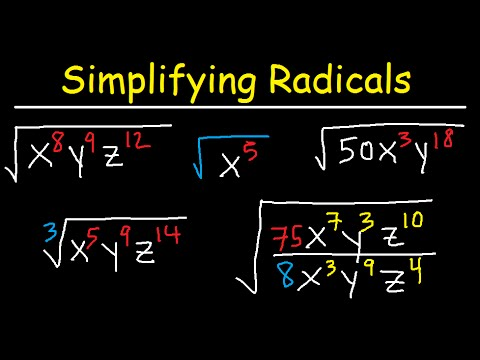By The Organic Chemistry Tutor

This algebra 1 & 2 video tutorial shows you how to simplify radicals with variables, fractions, and exponents that contains both square roots, cube roots, and variables such as x, y, and z. This video contains plenty of examples and practice problems.Here is a list of topics: 1. Simplifying Radical Expressions With Variables & Exponents 2. Simplifying Radicals With Fractions & Variables 3. Radicals With Square Roots & Cube Roots 4. Radical Expressions with X, Y, and Z 5. Absolute Value - Simplifying radical expressions# Qtr 1, Lesson 3: Rewriting Equations and Formulas

##By Stan Lisle

A lesson describing how to rewrite algebraic expressions with multiple variables and solve for any of the variables. Problems included to test the student's understanding.# Introduction to Random Variables - Khan Academy

##By Khan Academy

This video lecture series from Khan Academy on Probability includes Introduction to Probability, Permutations, Combinations, Probability using Combinations, Conditional probability, Introduction to Random Variables, Probability Density Functions, Binomial Distribution, Expected Value, Expected value of Binomial Distribution, Poisson Process, Law of Large Numbers# Expressions and variables

##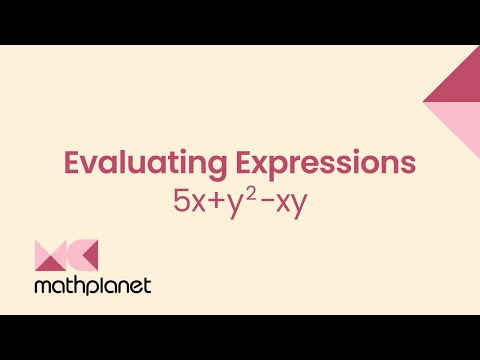By MathPlanetVideos

Evaluate the expression when x=4 and y=3 5x+y2−xy# Expressions with unknown variables 2

##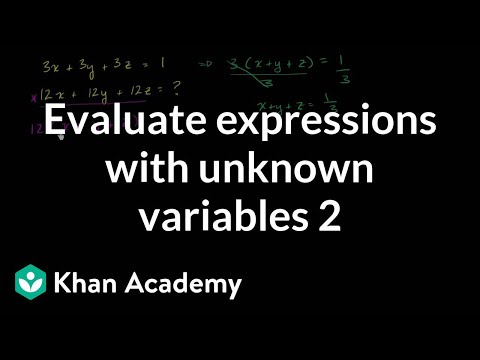By Khan Academy

A few more examples where Sal evaluates expressions with multiple unknown variables.# Expressions with unknown variables

##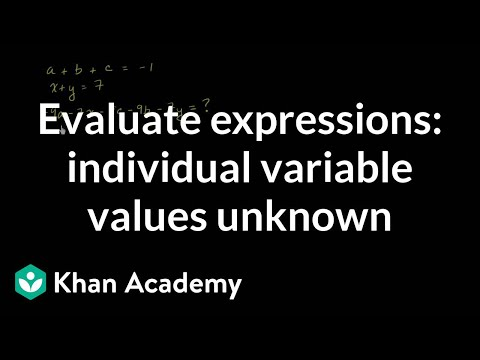By Khan Academy

Sal solved a few examples where we need to evaluate an expression, but we don't know the individual variable values.# Graphing - Independent Dependent Variables

##By MrG Lewis

Learn how to graph independent and dependent variables in this video from Mr G Lewis# Example 3: Finding the square of a binomial with two variables | Algebra I | Khan Academy

##By Khan Academy

This Khan Academy video offers more information on special products and contains a few samples with a step-by-step walkthrough on these new concepts.# Example 3: Finding the square of a binomial with two variables | Algebra I | Khan Academy

##By Khan Academy

This Khan Academy video offers more information on special products and contains a few samples with a step-by-step walkthrough on these new concepts.# Example 6: Subtracting polynomials with two variables | Algebra I | Khan Academy

##By Khan Academy

Example 6: Subtracting polynomials with two variables | Algebra I | Khan Academy# Composing expressions

##By MathPlanetVideos

Write an expression that describes the situation: Anna and her parents are going to the movies. Each ticket costs \$8 and Anna also wants popcorn which is an additional \$3. How much did they pay.# Mean and Expected Value of Discrete Random Variables

##By statslectures

This video summarizes for us in less than two minutes how to find the expected value for a discrete random variable. In order to apply this to empirical data, form a histogram from your data and use that to determine relative frequencies.# Independent and Dependent Variables - YourTeacher.com

##By yourteachermathhelp

This video briefly explains the difference between an independent and dependent variable using the example of the length of your hair based on how many days it has been since you got a hair cut.# Evaluating Expressions with Two Variables - Khan Academy

##By Khan Academy

Sal Khan shows the viewer how to evaluate an expression with two variables.# Expressions of Quantity (Part 1)

##By Smrt English

This video introduces count and uncount/noncount nouns and presents how to use the expressions "much" and "many".# Expressions of Quantity (Part 2)

##By Smrt English

This lesson presents when to use popular expressions of quantity such as "lots of, alot of, a few, a little"# Evaluating Expressions

##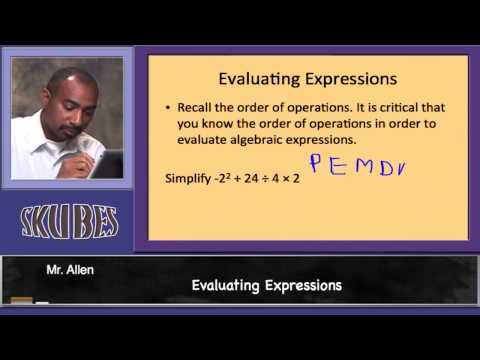By Skubes Ed

Interpret expressions that represent a quantity in terms of its context. Interpret parts of an expression, such as terms, factors, and coefficients.# Linear Equations in Two Variables

##By Meritnation

YouTube presents Linear Equations in Two Variables, an educational video resource on math.# Evaluate expressions

##By MathPlanetVideos

Evaluate the following expression for x = 2, y = 5 and z = 4 4x+(7−z)−6y# Simplify expressions

##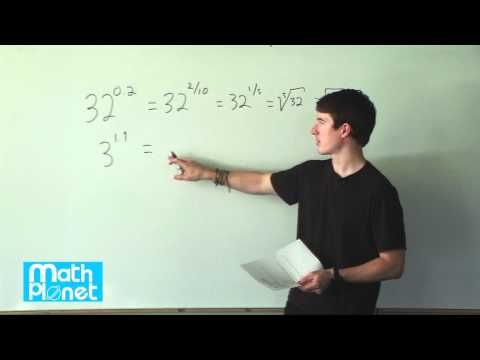By MathPlanetVideos

How can we rewrite 320,2?